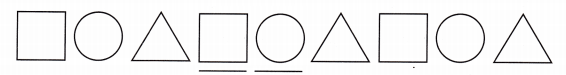# McGraw Hill Math Grade 4 Chapter 12 Lesson 9 Answer Key Problem Solving: Finding Patterns

Practice the questions of McGraw Hill Math Grade 4 Answer Key PDF Chapter 12 Lesson 9 Problem Solving: Finding Patterns to secure good marks & knowledge in the exams.

## McGraw-Hill Math Grade 4 Answer Key Chapter 12 Lesson 9 Problem Solving: Finding Patterns

Solve

Question 1.
Jenny draws this pattern.What shapes will complete the pattern?Explanation:
The shapes in the pattern are square, circle and triangle
So, the missing shapes are square and a circle.

Question 2.
Nasif starts with a number. He adds 920, multiplies by 3, subtracts 1,201, and then divides by 7. The result is 239. What number did Nasif start with?
38

Explanation:
Reverse all operations to find the number
Nasif starts with a number. He adds 920, multiplies by 3, subtracts 1,201, and then divides by 7
The result is 239
Multiply 239 by 7, 239 x 7 = 1673
Add 1201 to 1673, 1673 + 1201 = 2874
Divide 2874 with 3, 2874/3 = 958
Subtract 920 from 958, 958 – 920 = 38
So, Nasif started with the number 38.

Question 3.
A college teacher is reading reports from her students. The first report is 3 pages long. The second report is 6 pages long. The third report is 9 pages long. The fourth report is 12 pages long. If the pattern continues, how many pages will be in the eighth report?
24 pages

Explanation:
A college teacher is reading reports from her students
The first report is 3 pages long
The second report is 6 pages long
The third report is 9 pages long
The fourth report is 12 pages long
The pattern is 3, 6, 9, 12
Its is multiple of 3
So, the pattern will be 3, 6, 9, 12, 15, 18, 21, 24
The eighth report is 24 pages long.

Scroll to Top